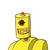# 5. What is the area of a triangle whosebase is 18.2 cm and height is 7.5cm?​

5. What is the area of a triangle whose
base is 18.2 cm and height is 7.5cm?​

### 1 thought on “5. What is the area of a triangle whose<br />base is 18.2 cm and height is 7.5cm?​”

1.## Given:–

What is the area of a triangle whose base is 18.2 cm and height is 7.5 cm?

## ToFind:–

Area of triangle, whose base and height both are given.

## Note:–

Area of triangle ( when base and height is given = ½ × ( base × height )

First bracket should be solved

## Solution:–

### Base=18.2cm,height=7.5cm,sonow~

Area of triangle = [tex] ½ × ( 18.2 cm × 7.5 cm ) [/tex]

Area of triangle = [tex] ½ × 136.50 cm² [/tex]